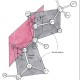A more detailed discussion of these same topics is now available here.

Introduction

Let’s consider the relative humidity we have right now in Venice, Italy: 97% with a temperature of 8°C and a pressure of 755 mmHg. Pretty humid, right? What about a warm place, let’s say Buenos Aires, in Argentina? Right now they have a relative humidity of 55%, at a temperature of 23° and a pressure of 760 mmHg. Now, which place is more humid, between these two? In other words: in which of these two places does the same volume of air contain the biggest amount of water? Are you sure you know the answer?

Some definitions and a formula

Relative humidity is defined as follows$RH\;=\;100\cdot\frac{e'}{e'_w}$

where${e'}$ is the partial pressure of water vapor in the air and$e'_w$ is the saturation vapor pressure with respect to water (the vapor pressure in the air in equilibrium with the surface of the water). We then have$e'_w(p,t)\;=\;(1.0016\;hPa+3.15\cdot 10^{-6} p-\frac{0.074}{p}\;hPa^{2}) 6.112\cdot e^{\frac{17.62t}{243.12+t}}$

Here and in what follows we express pressure in hPa, with$hPa\;=\;10^2 Pa$, while$t$ is the temperature in °C and$T$ is the temperature in$K$, with$T\;=\;273.15+t$. Absolute humidity is simply given by${\rho}_v\;=\;\frac{m_v}{V}$

where$m_v$ is the mass of vapor and$V$ is the total volume occupied by the mixture (Ref, chapter 4).

Physics of gasses

From the law of perfect gasses and considering that, according to Dalton’s law, in a moisture, the partial pressure of each gas is the pressure it would have if it occupied the whole volume (Ref), we can also write$e'\;=\;m_v\cdot R_v\frac{273,15 + t}{V}$

with$R_v\;=\;4.614\cdot\frac{hPa\cdot m^3}{kg\cdot K}$. Then, by substituting$e'$ and$m_v$ in${\rho}_v$, we have${\rho}_v\;=\;\frac{RH}{100\cdot R_v\cdot (273.15+t)}(1.0016\;hPa+3.15\cdot 10^{-6} p-\frac{0.074}{p} \;hPa^{2}) 6.112\cdot e^{\frac{17.62t}{243.12+t}}$

At the end of this post, you find a simple code in Octave that calculates this formula and the plot.

If we apply now the equation for${\rho}_v$ to the environmental parameters relative to Venice, we find that one$m^3$ of air contains 8 grams of water; if we repeat the same calculation for Buenos Aires we find that the same volume of air contains 11 grams of water. In other words, today Venice is less humid than Buenos Aires in the same period if we refer to the content of water in the air.

In the diagram below you can see the plot of absolute humidity as a function of the temperature, for three values of relative humidity. So, for instance, 20$\frac{g}{m^3}$ of water corresponds to an RH of 90% at a temperature of 24°C and to an RH of only 50% at a temperature of 35°C. The reason for this, beyond the mathematical formulae, is obvious: when the temperature of the air decreases, the ability of the moisture to retain water as vapor decreases accordingly.

Conclusion

Relative humidity is relevant for the subjective perception of heat (the more the relative humidity, the more difficult it is sweating for human beings, then the higher it is the perception of heat since we use sweating for cooling down). But if we are interested in the interaction of air and our lungs, for instance, relative humidity might be completely misleading and absolute humidity is likely the parameter to be considered (R).

The script

% file name = absolute_humidity
% it calculates the absolute humidity given the relative humidity and the temperature
clear all
% constant for water vapour (J/kg*K)
Rv = 461.4;
% relative humidity (%), temperature (°C), pressure (mmHg)
RH = 55
t = 23
pHg = 760
% conversion to Pa (1 mmHg = 133.322365 Pa)
p = pHg*133.322
% conversion to hPa=100Pa
p = p/100;
Rv = 4.614;
% absolute humidity (kg/m^3)
AH = (RH/(100*Rv*(273.15+t)))*(1.0016+3.15*10^(-6)*p-0.074/p)*6.112*e^(17.62*t/(243.12+t))
% we now fix the RH and plot AH for variuos values of t
RH = 50
pHg = 760
p = pHg*133.322;
p = p/100;
for i=1:30
t2(i)=i+5;
AH2(i) = 1000*(RH/(100*Rv*(273.15+t2(i))))*(1.0016+3.15*10^(-6)*p-0.074/p)*6.112*e^(17.62*t2(i)/(243.12+t2(i)));
endfor
plot (t2, AH2,’-k’,’LineWidth’,3)
grid on
grid minor
hold on
RH = 70
for i=1:30
t2(i)=i+5;
AH2(i) = 1000*(RH/(100*Rv*(273.15+t2(i))))*(1.0016+3.15*10^(-6)*p-0.074/p)*6.112*e^(17.62*t2(i)/(243.12+t2(i)));
endfor
plot (t2, AH2,’-r’,’LineWidth’,3)
hold on
RH = 90
for i=1:30
t2(i)=i+5;
AH2(i) = 1000*(RH/(100*Rv*(273.15+t2(i))))*(1.0016+3.15*10^(-6)*p-0.074/p)*6.112*e^(17.62*t2(i)/(243.12+t2(i)));
endfor
plot (t2, AH2,’-b’,’LineWidth’,3)
xlabel (‘temperature (°C)’)
ylabel (‘absolute humidity (g/{m^3})’)
legend (‘AH for RH = 50%’,’AH for RH = 70%’,’AH for RH = 90%’, ‘location’, ‘NORTHWEST’ )

The equations of this blog post were written using$\LaTeX$.

## 3 thoughts on “Relative Humidity is misleading”

1.Veronica says:

Ciao Paolo! Mi chiamo Veronica, vivo a Buenos Aires, il mio italiano e povero, scusi!
Tengo EM/SFC en grado moderado a severo hace 6 años, los inviernos son muy malos para mi, estoy encamada el 80 o 90% del dia. En la primavera obtengo usualmente una mejoria, paso de severa a moderada.
He leido que tambien mejoras con el cambio de clima, yo no sé si es la radiación UV o la temperatura, la presion atmosferica o la humedad? Yo también empeoro muchisimo cuando llueve, y cuando está a punto de llover.
Tienes alguna teoría acerca de esto?
Grazie e saluti!

Liked by 1 person

2.Paolo Maccallini says:

¡Hola Verónica!

No sé cuál es el parámetro ambiental que me hace mejorar en verano, todavía. Ahora creo que la humedad absoluta puede ser importante, pero no soy seguro.

Estoy estudiando este aspecto.

¡Gracias por escribirme!

Like

1.Veronica says:

Grazie Paolo, questa malattia è così misteriosa… Siempre te leo, me parecen muy interesantes tus reflexiones, un abrazo!

Liked by 1 person